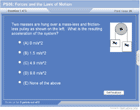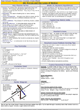How to Learn in 24 Hours?The Rapid Learning Movie

 Need Help? M-F: 9am-5pm(PST): Toll-Free: (877) RAPID-10 US Direct: (714) 692-2900 Int'l: 001-714-692-2900 24/7 Online Technical Support: The Rapid Support Center Secure Online Order:Got Questions? Frequently Asked Questions
 Need Proof? Testimonials by Our Users
 Trustlink is a Better Business Bureau Program. Rapid Learning Center is a fivr-star business. External TrustLink Reviews

 Rapid Learning Courses: MCAT in 24 Hours (2015-16) USMLE in 24 Hours (Boards) Chemistry in 24 Hours Biology in 24 Hours Physics in 24 Hours Mathematics in 24 Hours Psychology in 24 Hours SAT in 24 Hours ACT in 24 Hours AP in 24 Hours CLEP in 24 Hours DAT in 24 Hours (Dental) OAT in 24 Hours (Optometry) PCAT in 24 Hours (Pharmacy) Nursing Entrance Exams Certification in 24 Hours eBook - Survival Kits Audiobooks (MP3)

 Tell-A-Friend: Have friends taking science and math courses too? Tell them about our rapid learning system.Home »  Physics »  High School Physics

Forces and the Laws of Motion

 Topic Review on "Title": Newton’s 1st law: Inertia, the tendency to continue in a given state. Newton’s 2nd law: The acceleration of an object is directly proportional to the net force, and inversely proportional to the mass. F=ma Newton’s 3rd law: For every force, there is an equal and opposite force. One Newton: The force need to accelerate 1kg at 1 m/s/s. Normal Force: A reaction force pointing perpendicular to the surface. Equilibrium: When all the forces on an object balance out, or cancel out, the object has a net force of 0. Static Equilibrium: When an object is in equilibrium, and not moving, this is called static equilibrium. Dynamic Equilibrium: An object moving at a constant velocity; no net force acting on it. Friction: A force that always opposes motion. Coefficient of friction: The ratio between the frictional force and the normal force.

Rapid Study Kit for "Title":
 Flash Movie Flash Game Flash Card Core Concept Tutorial Problem Solving Drill Review Cheat Sheet"Title" Tutorial Summary : This tutorial will describe Newton’s three laws of motion.  These simple, but very powerful statements can describe a great deal of the behavior of motion.  Additionally, the concept of equilibrium will be discussed.  This is a situation where the net force on an object is zero.  In situations like this, forces such as friction and air resistance may come into play.  Both of these forces tend to slow, or resist any movement.  Finally, dynamics problems will be addressed.  Here, there is no equilibrium.  There is a net force exerted on something that causes an acceleration.

 Tutorial Features: Specific Tutorial Features: • Graphically see force diagrams with animated components, especially the inclined plane problems. Series Features: • Concept map showing inter-connections of new concepts in this tutorial and those previously introduced. • Definition slides introduce terms as they are needed. • Visual representation of concepts • Animated examples—worked out step by step • A concise summary is given at the conclusion of the tutorial.

 "Title" Topic List: Newton’s Laws Inertia Net Force Action and reaction forces Equilibrium Dynamic equilibrium Static equilibrium Resisting Forces Friction Air resistance Dynamics Problems

See all 24 lessons in high school  physics, including concept tutorials, problem drills and cheat sheets:
Teach Yourself High School Physics Visually in 24 Hours